# 前端:从零破解一款轻量级滑动验证码

163次阅读  |  发布于6月以前

## 思路讲解

### 打开页面

1. 根据用户标识，从后台获得验证码图片
2. 监听鼠标事件并回传后台
3. 后台判断事件的真伪，回传验证结果

Puppeteer 是一个通过 DevTools 协议来控制浏览器行为的库，只需编写不多的代码，就可以操作真实的浏览器搞定诸如爬虫、自动化测试、网页性能分析、浏览器扩展测试等功能。使用起来比较方便，它文档挺全的，根据文档，打开页面前需要启动一个浏览器实例，然后调用 newPage 方法创建一个新页面。核心代码如下。

``````const puppeteer = require('puppeteer')

puppeteer.launch().then(async browser => {
const page = await browser.newPage()
await page.goto('http://h5.dooring.cn/slider-vertify/vertify')
})``````打开页面测试

1. 等待验证码图片加载完毕
2. 移动鼠标到滑块位置
3. 按下鼠标
4. 移动鼠标到缺口位置
5. 松开鼠标
6. 等待结果返回

### 判断位置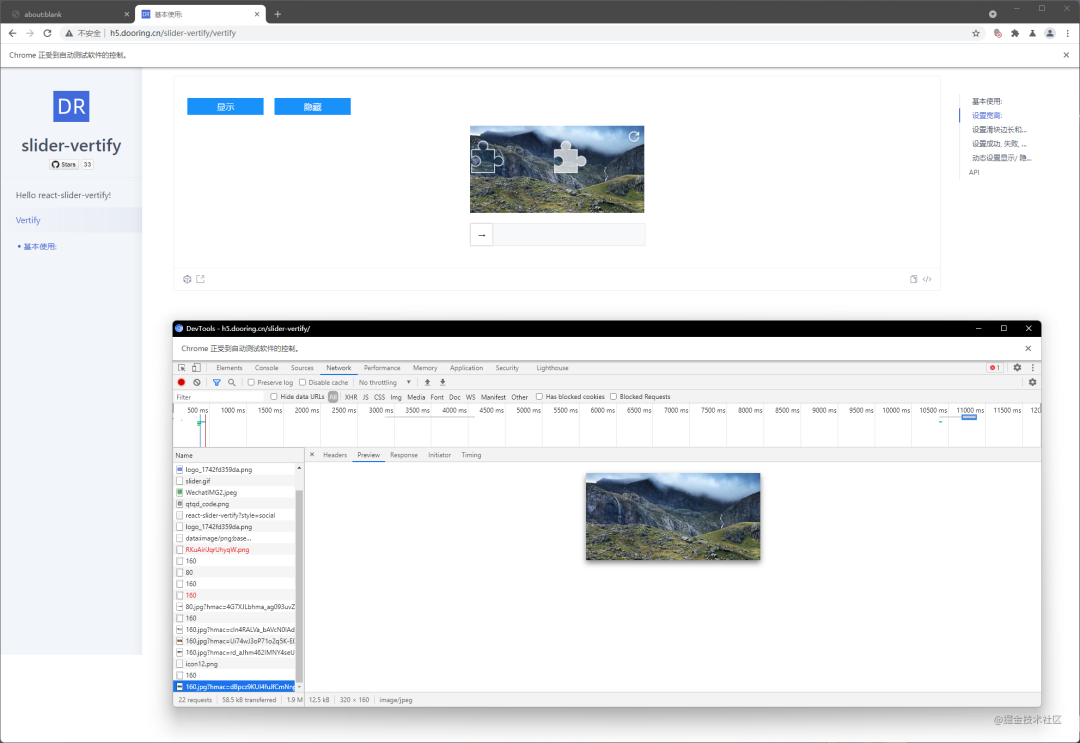找到请求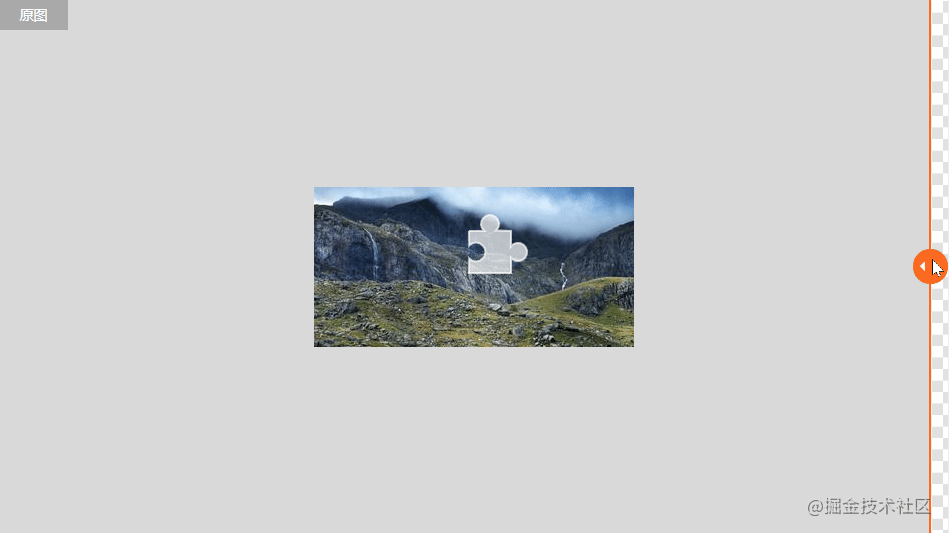阿里云图像分割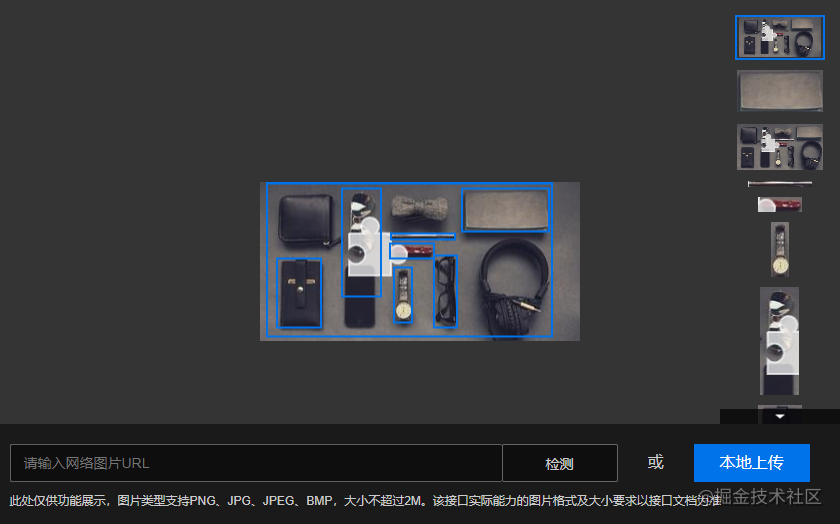百度云图像主体识别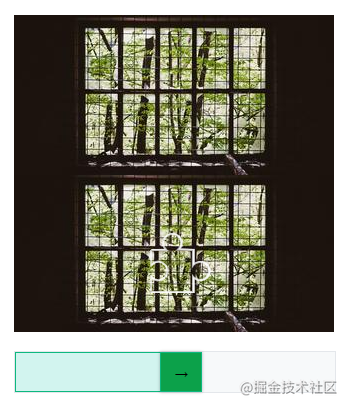和原图进行比对

``````while (left <= maxOffset) {

/* 使用 CSS left 属性控制悬浮的滑块的偏移量 */

await page.evaluate(async (\$sliderFloat, left) => {
\$sliderFloat.setAttribute('style', `left: \${left}px`)
}, \$sliderFloat, left)

/* 截图并和原图进行比对，把结果存到 results 数组里 */

const \$panel = await page.\$('#Vertify-demo-4 .canvasArea')
const panelImgBase64 = await \$panel.screenshot({
type: 'jpeg'
})
const compareRes = await rembrandt({
imageA: panelImgBase64,
imageB: rawImage
})
results.push({
left,
diff: compareRes.differences
})

left += 1
}
``````通过CSS控制位移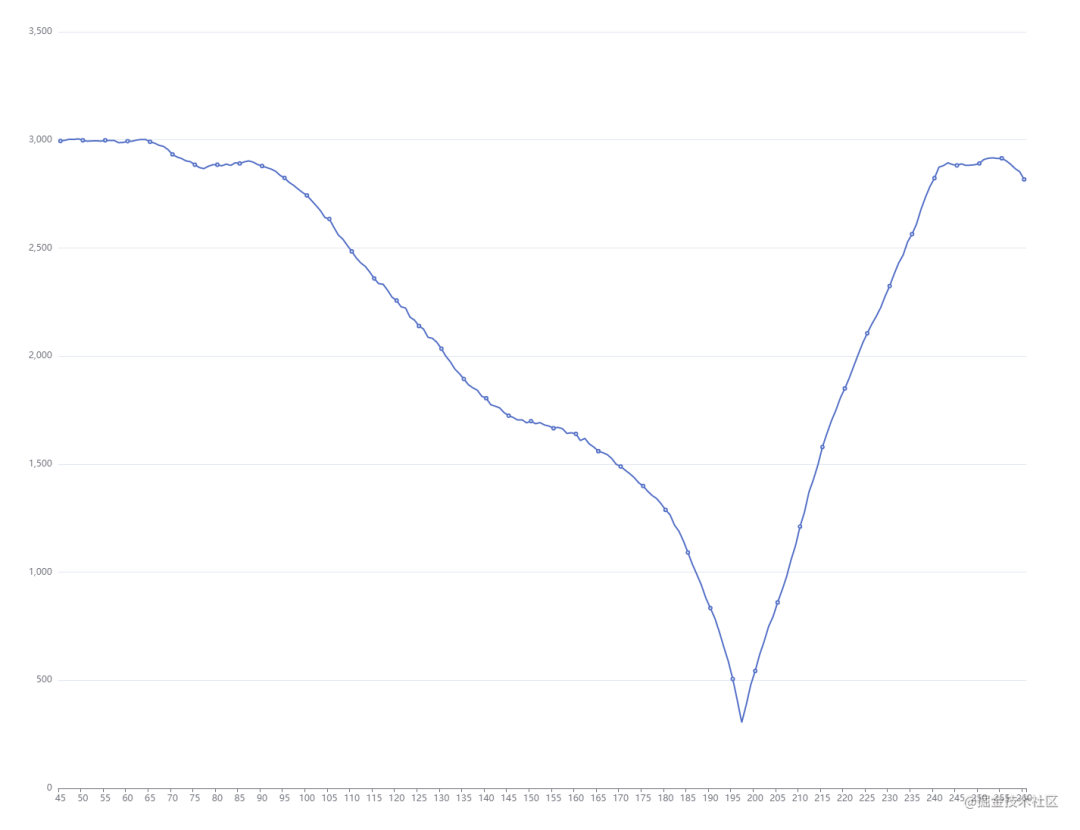图像比对结果

1. 把元素缩小，复制多份，平铺开来展示；这样只要截一次，然后再裁剪、比对就好。
2. 放大步长，比方说先每次平移 15px，找到局部最优解，然后在局部最优解附近再回到平移 1px 的方案找最优解。
3. 因为图片比对的结果类似“V”字，“V”字右半边其实是可以不用再计算的。

``````// 图片缺口是不会给挖在初始附近的，
// 所以 left 从 45 像素开始计算可以节约不少计算量，
let left = 45;
const max15Offset = 265;
const res15px = [];
while (left <= max15Offset) {
await setLeft(left);
const compareRes = await compare();
res15px.push({
left,
diff: compareRes.differences,
});
left += 15;
}
``````

``````const min15pxDiff = Math.min(...res15px.map((x) => x.diff));
const min15pxLeft = res15px.find((x) => x.diff === min15pxDiff).left;

left = min15pxLeft - 12;
const max2Offset = min15pxLeft + 8;
const res2px = [];
while (left <= max2Offset) {
await setLeft(left);
const compareRes = await compare();
res2px.push({
left,
diff: compareRes.differences,
});
left += 2;
}
``````

[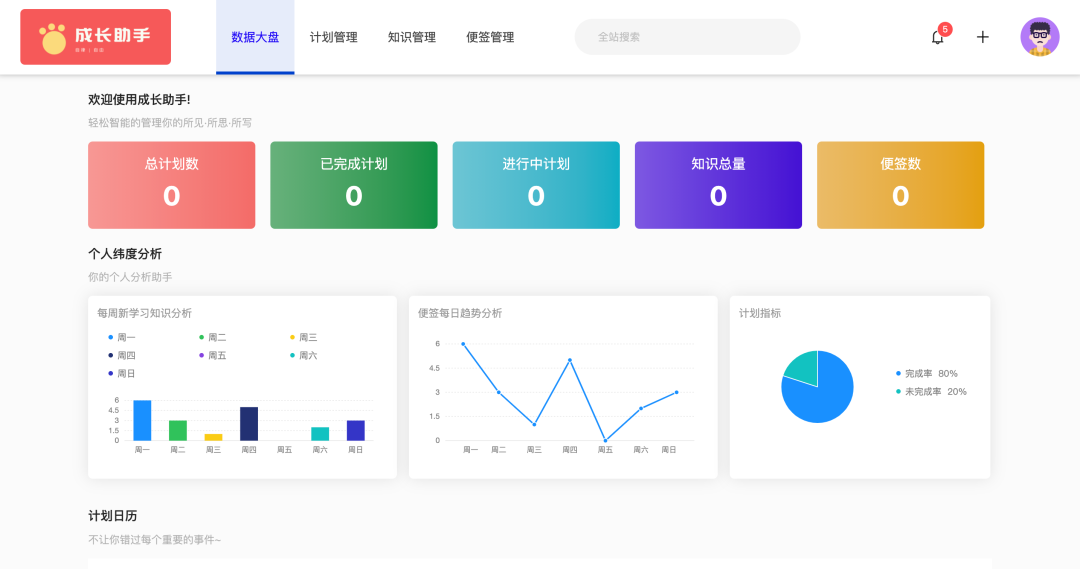]

### 移动鼠标

Puppeteer 已经提供了鼠标相关的接口，一共四个：mouse.click、mouse.down、mouse.move、mouse.up，分别是点击、按下、移动和松开。使用 mouse.move 可以直接把鼠标位置移动到一个特定的坐标上。假设我们现在从坐标（100,100）花大约 1s 把鼠标移动到 （200,200），可以使用循环实现。

``````const now = {
x: 100,
y: 100
}
const target = {
time: 1000,
x: 200,
y: 200,
}
const steps = 10
const step = {
x: Math.floor((target.x - now.x) / steps),
y: Math.floor((target.y - now.y) / steps),
time: target.time / steps
}
while (now.x < target.x) {
await sleep(step.time)
now.x += step.x
now.y += step.y
await page.mouse.move(now)
}
``````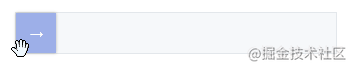鼠标轨迹不稳定

• 鼠标 Y 轴位置总是在变
• 鼠标 X 轴位置会滑过头

``````// 获得一个随机的偏移量
const getRandOffset = (enableNegative = true, max = 3) => {
const negative = enableNegative
? (Math.random() < 0.5) ? -1 : 1
: 1
return Math.floor(Math.random() * max) * negative
}

// 先滑过头十几像素，然后再花 100 毫秒的时间往回滑到正确位置
const targets = [
{
time: 1000,
x: 200 + getRandOffset(false, 15),
y: 200 + getRandOffset(false, 15),
steps: 10
},
{
time: 100,
x: 200,
y: 200,
steps: 3
}
]

// 注意这里用 for await 循环把 targets 串起来执行
for await (const target of targets) {
const step = {
x: Math.floor((target.x - now.x) / target.steps),
y: Math.floor((target.y - now.y) / target.steps),
time: target.time / target.steps,
}
let gap
while (gap = Math.abs((target.x - now.x)), gap > 0) {
await sleep(step.time)
// 最后一步就直接滑动到位，不需要随机数了
const inOneStep = Math.abs(target.x - now.x) <= Math.abs(step.x);
if (inOneStep) {
now.x = target.x;
now.y = target.y;
} else {
now.x += step.x + getRandOffset();
now.y += step.y + getRandOffset();
}
moveMouseTo(now)
}
}
``````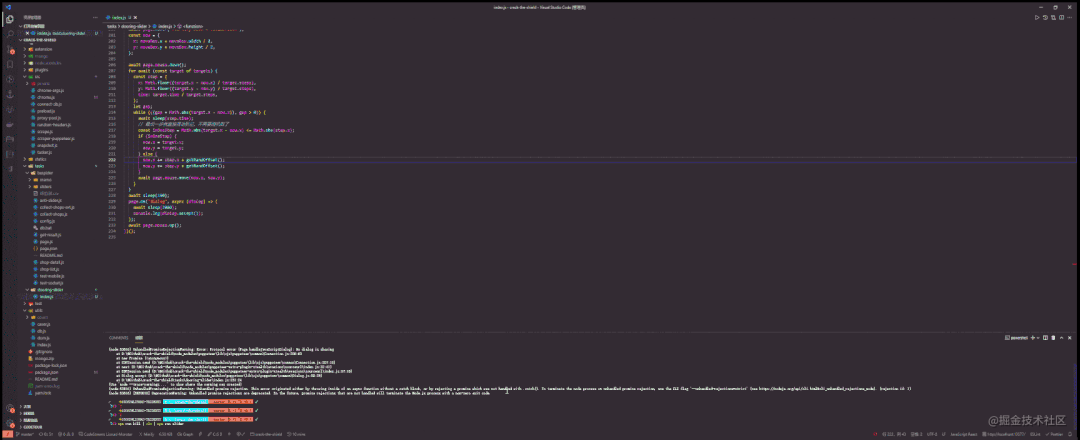最终效果（加速）